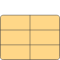# BridgmansTables

Calculates all entries in Bridgmans tables if first seven variables given# Information

This information is part of the Modelica Standard Library maintained by the Modelica Association.

Important: the phase equilibrium conditions are not yet considered. this means that Bridgman's tables do not yet work in the two phase region. Some derivatives are 0 or infinity anyways. Idea: Do not use the values in Bridgmans table directly, all derivatives are calculated as the quotient of two entries in the table. The last letter indicates which variable is held constant in taking the derivative. The second letters are the two variables involved in the derivative and the first letter is always a d to remind of differentiation.

```Example 1: Get the derivative of specific entropy s w.r.t. Temperature at
constant specific volume (between identical to constant density)
constant volume  --> last letter v
Temperature      --> second letter T
Specific entropy --> second letter s
--> the needed value is dsv/dTv
Known variables:
Temperature T
pressure p
specific volume v
specific inner energy u
specific enthalpy h
specific entropy s
specific Helmholtz energy f
specific gibbs enthalpy g
Not included but useful:
density d
In order to convert derivatives involving density use the following
rules:
at constant density == at constant specific volume
ddx/dyx = -d*d*dvx/dyx with y,x any of T,p,u,h,s,f,g
dyx/ddx = -1/(d*d)dyx/dvx with y,x any of T,p,u,h,s,f,g
Usage example assuming water as the medium:
model BridgmansTablesForWater
extends ThermoFluid.BaseClasses.MediumModels.Water.WaterSteamMedium_ph;
Real derOfsByTAtConstantv "derivative of sp. entropy by temperature at constant sp. volume"
ThermoFluid.BaseClasses.MediumModels.Common.BridgmansTables bt;
equation